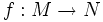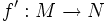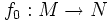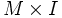# Smooth homotopy theorem

1. Suppose$f:M \to N$ is a continuous map between differential manifolds. Then, there exists a smooth map$f':M \to N$ such that$f'$ is homotopy-equivalent to$f$
2. Suppose$F:M \times I \to N$ is a homotopy between smooth maps$f_0:M \to N$ and$f_1:M \to N$. Then, there exists a homotopy$F':M \times I \to N$ from$f_0$ to$f_1$ that is smooth as a map from$M \times I$ to$N$.